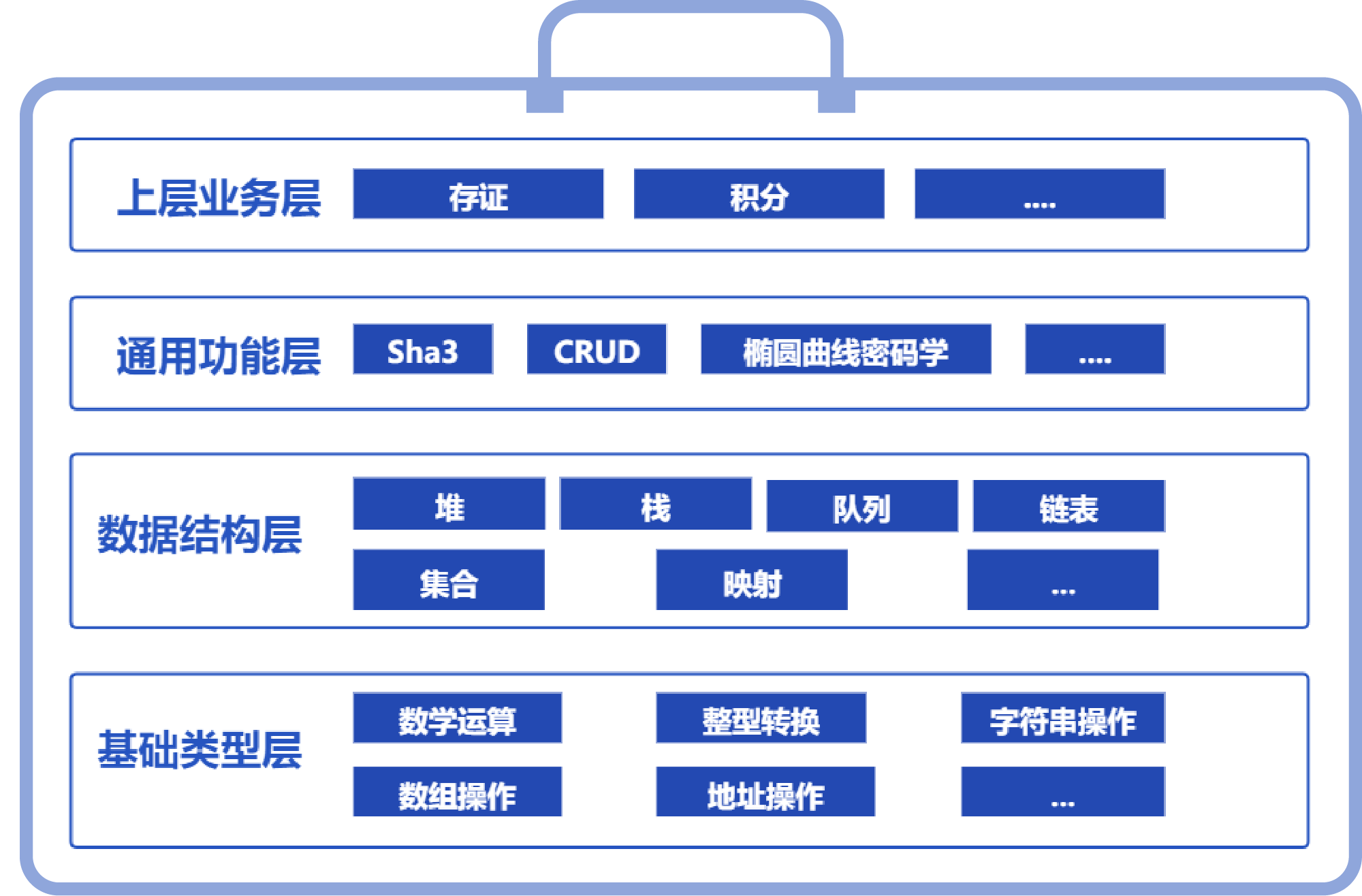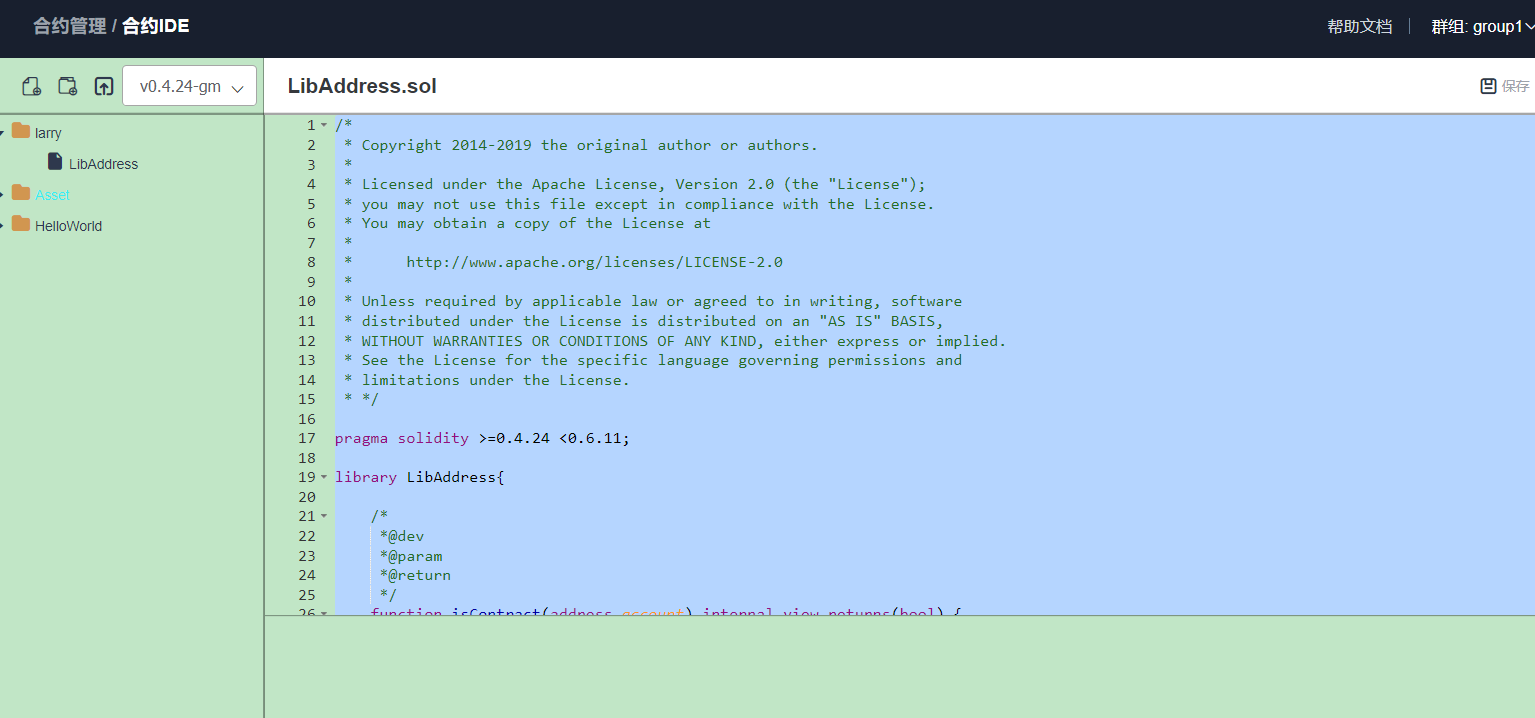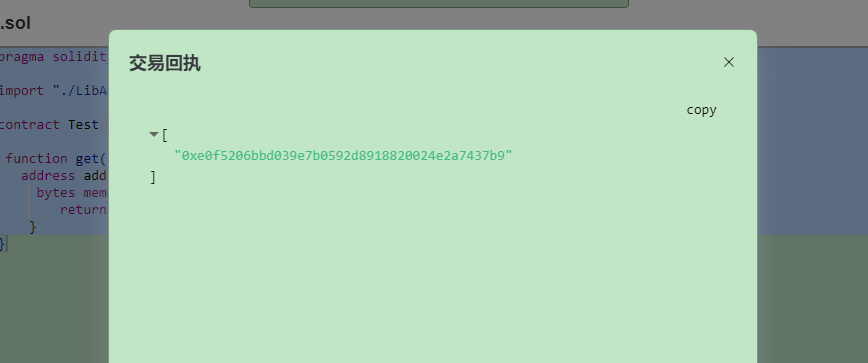# 一、智能合约库简介

SmartDev-Contract智能合约库是一个“麻雀虽小，五脏俱全”的智能合约的工具类库，通过Solidity的library封装，旨在帮助Solidity开发者提升开发体验，避免重复造轮子，让编写Solidity语言也可以如编写Python语言那样“丝滑顺畅”。

SmartDev-Contract智能合约库的每个合约文件都来自于微众银行区块链工程师的细致打磨，来自于实际使用场景的“聚沙成塔”，覆盖了业务场景开发中的各种“犄角旮旯”，是开发智能合约的“10倍工程师”的不二法门。# 二、痛点及解决方式

``````pragma solidity ^0.4.25;
contract Demo {
...
}``````测试代码：

``````pragma solidity ^0.4.24;

contract Test {

function get() public view returns (bytes memory) {
return bs;
}
}``````## 痛点一：计算可能溢出

SmartDev-Contract智能合约库提供了安全计算的代码类库。以uint256数据类型为例，LibSafeMathForUint256Utils提供了Uint256类型的相关计算操作，且保证数据的正确性和安全性，包括加法、减法、乘法、除法、取模、乘方、最大值、最小值和平均数等操作。其他的数值类型可以自行参考实现。

LibSafeMathForUint256Utils下载地址： https://gitee.com/WeBankBlockchain/SmartDev-Contract/blob/master/contracts/base_type/LibSafeMathForUint256Utils.sol 下载之后导入需要使用的sol文件中，然后就可以调用LibSafeMathForUint256Utils相关的方法，具体的如下所示：

1、加减乘除运算

``````function f() public view {
uint256 a = 25;
uint256 b = 20;
// a + b
// a - b
uint256 d = LibSafeMathForUint256Utils.sub(a,b);
// a * b
uint256 e = LibSafeMathForUint256Utils.mul(a,b);
// a/b
uint256 f = LibSafeMathForUint256Utils.div(a,b);
}``````

2、取模运算、乘方运算

``````function f() public view {
uint256 a = 25;
uint256 b = 20;
// a % b
uint256 c = LibSafeMathForUint256Utils.mod(a,b);
// a ^ b
uint256 d = LibSafeMathForUint256Utils.power(a,b);
}``````

3、最大值、最小值、平均数运算

``````function f() public view {
uint256 a = 25;
uint256 b = 20;
// max(a, b)
uint256 c= LibSafeMathForUint256Utils.max(a,b);
// min(a, b)
uint256 d = LibSafeMathForUint256Utils.min(a,b);
// average(a, b)
uint256 e = LibSafeMathForUint256Utils.average(a,b);
}``````

## 痛点二：转换不够便捷

LibConverter下载地址： https://gitee.com/WeBankBlockchain/SmartDev-Contract/blob/master/contracts/base_type/LibConverter.sol 下载之后导入需要使用的sol文件中，然后就可以调用LibConverter相关的方法，具体的如下所示：

1、数值类型向下转换，例如uint256转换为uint8。

``````function f() public view{
uint256 a = 25;
uint8 b = LibConverter.toUint8(a);
}``````

2、数值类型转bytes

``````function f() public view{
uint256 a = 25;
bytes memory b = LibConverter.uintToBytes(a);
}``````

3、bytes转数值类型

``````function f() public view{
bytes memory a = "25";
int b = LibConverter.bytesToInt(a);
}``````

``````address addr = 0xE0f5206BBD039e7b0592d8918820024e2a7437b9;

``````bytes memory bs = newbytes(20);

``````address addr = 0xE0f5206BBD039e7b0592d8918820024e2a7437b9;

``````string memory str="0xE0f5206BBD039e7b0592d8918820024e2a7437b9";

## 痛点三：数组操作不够丰富

SmartDev-Contract智能合约库基于动态数组的结构封装了“LibArrayForUint256Utils”的常用工具函数实现。开发者也可根据自身需要的数据结构，自行封装相关的工具类。 LibArrayForUint256Utils下载地址： https://gitee.com/WeBankBlockchain/SmartDev-Contract/blob/master/contracts/base_type/LibArrayForUint256Utils.sol 下载之后导入需要使用的sol文件中，然后就可以调用LibArrayForUint256Utils相关的方法，具体的如下所示：

1、添加不重复的元素

``````uint[] private array;
function f() public view {
array=new uint[](0);
// array: {2}
}``````

2、合并两个数组

``````uint[] private array1;
uint[] private array1;
function f() public view {
array1=new uint[](2);
array2=new uint[](2);
LibArrayForUint256Utils.extend(array1,array2);
// array1 length 4
}``````

3、对数组去重

``````uint[] private array;
function f() public view {
array=new uint[](2);
array=2;
array=2;
LibArrayForUint256Utils.distinct(array);
// array: {2}
}``````

4、对数组升序排序

``````uint[] private array;
function f() public view {
array=new uint[](3);
array=3;
array=2;
array=1;
LibArrayForUint256Utils.qsort(array);
// array: {1,2,3}
}``````

5、二分查找

``````uint[] private array;
function f() public view {
array=new uint[](3);
array=3;
array=2;
array=1;
uint256 key=3;
LibArrayForUint256Utils.binarySearch(array,key);
// array: {true, 1}
}``````

6、删除元素

``````uint[] private array;
function f() public view {
array=new uint[](3);
array=3;
array=2;
array=1;
LibArrayForUint256Utils.removeByValue(array,2);
// array: {3, 1}
}``````

## 痛点四：不提供字符串内置操作

1、获取字符串长度

``````pragma solidity ^0.4.25;
import "./LibString.sol";
contract Test {
function f() public{
string memory str = "你好";
uint256 lenOfChars = LibString.lenOfChars(str);
uint256 lenOfBytes = LibString.lenOfBytes(str);
require(lenOfChars == 2);
require(lenOfBytes == 6);
}
}``````

2、大小写转换

``````pragma solidity ^0.4.25;
import "./LibString.sol";
contract Test {
function f() public view returns(string memory)  {
string memory c = LibString.toUppercase("abcd");// Expected to be ABCD
return c;
}
}``````

3、相等比较

``````pragma solidity ^0.4.25;
import "./LibString.sol";
contract Test {
function f() public view {
bool r = LibString.equal("abcd","abcd");//Expected to be true
require(r);
}
}``````

4、字符串前缀比较

``````pragma solidity ^0.4.25;
import "./LibString.sol";
contract Test {
function f() public view {
bool r = LibString.startWith("abcd","ab");//Expected to be true
require(r);
}
}``````

## 痛点五：高级数据结构不完备

Mapping映射

https://gitee.com/WeBankBlockchain/SmartDev-Contract/blob/master/contracts/data_structure/LibBytesMap.sol

``````pragma solidity ^0.4.25;
import "./LibBytesMap.sol";

contract Test {
using LibBytesMap for LibBytesMap.Map;
LibBytesMap.Map private map;
event Log(bytes key, uint256 index);
function f() public {
string memory k1 = "k1";
string memory k2 = "k2";
string memory k3 = "k3";
string memory v1 = "v1";
string memory v2 = "v2";
string memory v3 = "v3";
map.put(bytes(k1),bytes(v1));
map.put(bytes(k2),bytes(v2));
map.put(bytes(k3),bytes(v3));
// 开始迭代
uint256 i = map.iterate_start();
while(map.can_iterate(i)){
emit Log(map.getKeyByIndex(i), i);
i = map.iterate_next(i);
}
}
}``````

SmartDev-Contract智能合约库依托动态数组和mapping，实现了一个基础的set集合。此外，由于Solidity不支持泛型机制，开发者可以参考此工具，实现其他元素的set集合。

``````pragma solidity ^0.4.25;

contract Test {
event Log(uint256 size);
//添加元素；
// {1}
// 查询set容器数量
require(size == 1);
// 获取指定index的元素
// 返回set中所有的元素
// {0x1}
// 判断元素是否存在
require(contains== true);
// 删除元素
}
}``````

# 总结

• 发表于 2021-04-21 10:54
• 阅读 ( 1576 )
• 学分 ( 35 )
• 分类：FISCO BCOS